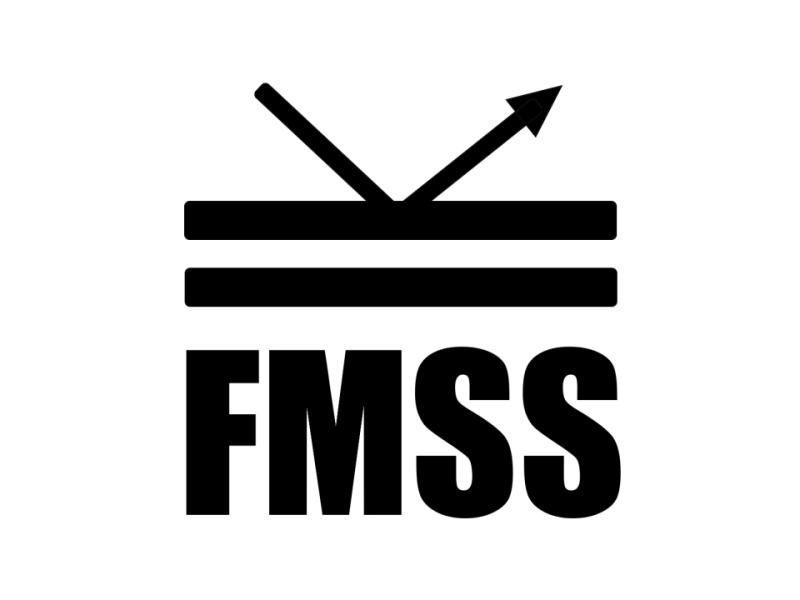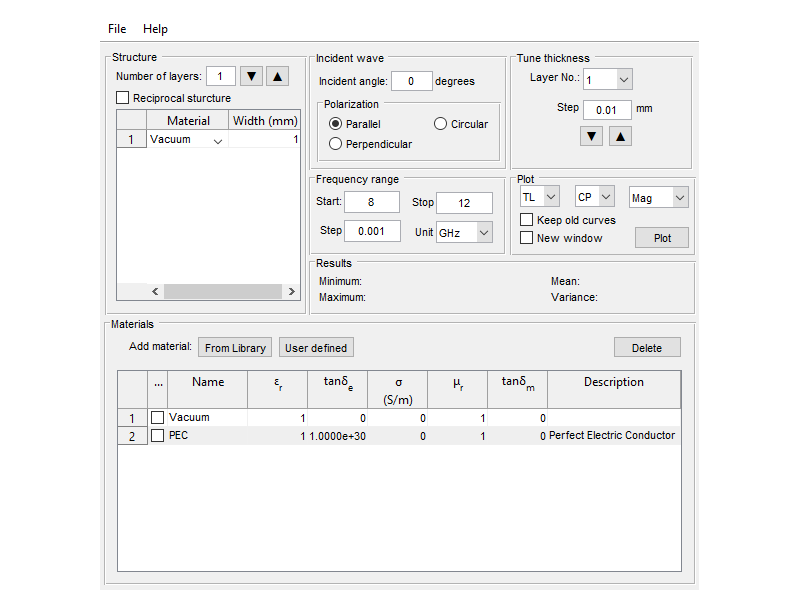# Flat Multilayer Structure Simulator (FMSS)Flat Multi-layer Structure Simulator (FMSS) is a software for calculation of reflection and transmission coefficients of a plane wave incident on a flat multilayered structure. The reflection and transmission coefficients are plotted in terms of frequency in the range specified by the user.

The material of each layer in the stack-up can be selected among the materials which have been defined in the software. The software has a library including a few common materials but the user can also define new materials and assign them to layers. The user can save the defined materials as well as the stack-up and load them again to use.

FMSS can be used in different parts of electromagnetic spectrum. In Radio Frequency (RF), FMSS can be used for the following applications:

• Calculation of transmission loss for estimating the electromagnetic performance of radomes including single layer and sandwich radomes
• Calculation of return loss for estimating the electormagnetic performance of absorbers and reduction of Radar Cross Section (RCS)

In optical frequencies, FMSS can be used for estimation of reflectance and transmittance of thin films and multilayered structures e.g. dielectric mirrors.

It is noted that FMSS assumes:

• All the incident, reflected, and transmitted rays are electromagnetic uniform plane waves.
• All the layers are flat and have infinite dimensions.
• The materials of layers are simple, i.e. electromagnetic properties of materials are isotropic, linear, non-dispersive, and homogeneous.

Flat Multi-layer Structure Simulator (FMSS) is a software for calculation of reflection and transmission coefficients of a plane wave incident on a flat multilayered structure. The reflection and transmission coefficients are plotted in terms of frequency in the range specified by the user.

The material of each layer in the stack-up can be selected among the materials which have been defined in the software. The software has a library including a few common materials but the user can also define new materials and assign them to layers. The user can save the defined materials as well as the stack-up and load them again to use.

FMSS can be used in different parts of electromagnetic spectrum. In Radio Frequency (RF), FMSS can be used for the following applications:

• Calculation of transmission loss for estimating the electromagnetic performance of radomes including single layer and sandwich radomes
• Calculation of return loss for estimating the electormagnetic performance of absorbers and reduction of Radar Cross Section (RCS)

In optical frequencies, FMSS can be used for estimation of reflectance and transmittance of thin films and multilayered structures e.g. dielectric mirrors.

It is noted that FMSS assumes:

• All the incident, reflected, and transmitted rays are electromagnetic uniform plane waves.
• All the layers are flat and have infinite dimensions.
• The materials of layers are simple, i.e. electromagnetic properties of materials are isotropic, linear, non-dispersive, and homogeneous.